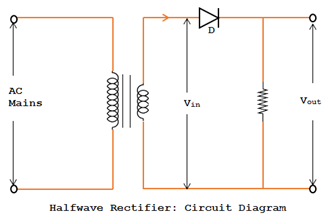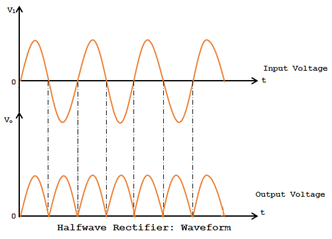# Half Wave Rectifier

### Rectifiers:Electronics circuits need DC voltage for biasing. The commercial power supply for consumer is in the form of AC. Hence there is a need to convert AC voltage to DC voltage.

A circuit which converts AC voltage onto DC voltage is called rectifier. The unidirectional conducting property of a diode is used in rectification.

### Diode Rectifiers

For reason associated with economics of generation and transmission, the electric power available is usually an AC supply.

The supply voltage varies sinusoidally and has a frequency of 50 Hz. It is used for lightning, heating and electric motors.

But there are any applications where DC supply is needed. When such a DC supply is required, the mains AC supply is rectified by using diodes.
The following three rectifier circuits can be used:
1. Half Wave rectifier
2. Full wave Rectifier
3. Bridge Rectifier

### Half Wave Rectifier:

The fig below shows that the circuit diagram of half wave rectifier.
• It consists of a transformer, a diode and a load resistor. Depending on the output of DC voltage required, a transformer of proper turn’s ratio N1:N2 is selected.
• The primary coil of a transformer is connected to the AC mains and the secondary coil to a load resistance RL through the diode D. The voltage of the secondary coil of the transformer is the input for the rectifier.
• Let V=Vm sinωt be the instantaneous sinusoidal voltage of frequency (=50Hz) appearing at the secondary coil of the transformer.
• During the positive half-cycle of the input voltage, the diode D is forward biased and it conducts the current through RL. Since the diode offers negligible resistance in the forward bias, the entire secondary voltage of transformer appears across RL as output voltage.
• During the negative cycle of the sine wave, the diode D is reverse biased. Hence no current flows in the circuit and no voltage develops across RL.• Only the positive half cycle of the input appears across the load. The input AC voltage I converted into a pulsating DC voltage as shown in waveform in the figure. This process is called half wave rectification.
• The output voltage is unidirectional, pulsating and intermittent. The output voltage contains a DC component and an AC component known as ripple.

#### Peak Inverse Voltage:

The maximum negative voltage appearing across the diode when it is not conducting is called peak inverse voltage denoted by PIV.
PIV = Vm

#### Ripple Factor:

Ripple factor is defined as the ratio of the RMS value of the AC component of load current to the average value of load current. It is a measure of imperfection in the DC output.
Ripple Factor γ = RMS value of AC component / Average value of current
= Iac / Idc  = 1.21

#### Efficiency Rectification:

The efficiency of rectification is defined as the ration of the DC output power to the input AC power.
Rectified Efficiency = O/p DC power / Input AC power
= Pdc / Pac  ~   40.6%

#### Voltage Regulation:

The regulation is a measure of the variation of the DC output voltage as a function of the DC current and is defined as
For an ideal power supply the % regulation will be zero.

#### Disadvantage of Half Wave Rectifier:

• Since the ripple factor = 1.21 is greater than C value, the half wave rectifier is a poor device for the conversion of AC voltage into DC voltage.
• Efficiency of rectification is very low (= 0.406)
• It has a low transformer utilization factor.1 CommentsComments

#### Read Comment Policy We have Zero Tolerance to Spam. Chessy Comments and Comments with Links will be deleted immediately upon our review.

1.This comment has been removed by a blog administrator.